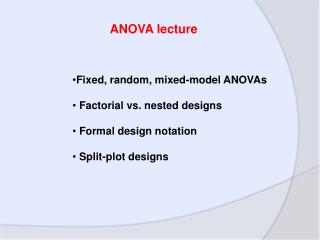# Fixed, random, mixed-model ANOVAs Factorial vs. nested designs Formal design notation - PowerPoint PPT PresentationDownload PresentationFixed, random, mixed-model ANOVAs Factorial vs. nested designs Formal design notation

Fixed, random, mixed-model ANOVAs Factorial vs. nested designs Formal design notationDownload Presentation## Fixed, random, mixed-model ANOVAs Factorial vs. nested designs Formal design notation

- - - - - - - - - - - - - - - - - - - - - - - - - - - E N D - - - - - - - - - - - - - - - - - - - - - - - - - - -
##### Presentation Transcript

1. ANOVA lecture • Fixed, random, mixed-model ANOVAs • Factorial vs. nested designs • Formal design notation • Split-plot designs

2. Goals • Describe your ANOVA design to a statistician (who can then help you analyse it). • Recognize three common types of ANOVA designs: • Factorial: fixed, randomized block • Nested • Split-plot • 3. For your reference: formulas for F tests for each

3. Factor – a variable of interest e.g. temperature Level – a particular value / state of a factor e.g. hot, cold In this example, temperature is a factor with two levels.

4. Fixed factor Either (1) The investigator chooses the levels of the factor for some purpose. Eg. Ambient CO2 vs. double CO2 OR (2) The levels used represent all possible levels. Eg. Biological sex: Male, female

5. Random factor The levels of the factor are chosen randomly from a universe of possible levels. Eg. We want to look at whether butterfly collectors differ in their diversity estimates for 4 plots. We select 5 collectors “randomly” from a village. Eg. We use three breeding lines of fruit flies as blocks in a genetics experiment. Blocks are typically random effects!

6. Formal notation Af6 is a fixed factor called A with 6 levels Br5 is a random factor called B with 5 levels

7. Group exercise (groups of 3) • Experimental design handout • Write out the factors and levels using formal notation

8. ANOVA Example: formal notation Example 1 Ecologists: Er10 Papers: Pf2 Example 2: Populations: Pr4 Herbivory: Hf2 Example 3: Light: Lf3 Nutrients: Nf3 Blocks: Br3

9. Fixed-effects ANOVA (Model I) • All factors are fixed • Random-effects ANOVA (Model II) • All factors are random • Mixed-model ANOVA (Model III) • Contains both fixed and random effects, e.g. randomized block!

10. Two-way factorial ANOVA How to calculate “F” Random effect (factors A & B random) Mixed model (A fixed, B random) Fixed effect (factors A & B fixed) Factor A MS A MS Error MS A MS A x B MS A MS A x B Factor B MS B MS A x B MS B MS Error MS B MS Error A x B MS A x B MS Error MS A x B MS Error MS A x B MS Error

11. Factorial design: All levels of one factor crossed by all levels of another factor, i.e. all possible combinations are represented. If you can fill in a table with unique replicates, it’s factorial! Double CO2 Ambient CO2 Pea plant Bean plant Corn plant

12. No fertilizer Nitrogen fertilizer Phosphorus fertilizer Strain A Strain B Strain C Strain D Strain E Strain F Nested design In this example, strain type is “nested within” fertilizer. Fertilizer is often called “group”, strain “subgroup” The nested factor is always random

13. Fertilizer O N P Strain A Strain B Strain C Strain D Strain E Strain F

14. Grand mean Variance: Group No fertilizer Nitrogen fertilizer Phosphorus fertilizer Strain A Strain B Strain C Strain D Strain E Strain F

15. Grand mean Variance: Group No fertilizer Nitrogen fertilizer Phosphorus fertilizer Variance: Subgroup within a group Strain A Strain B Strain C Strain D Strain E Strain F

16. Grand mean Variance: Group No fertilizer Nitrogen fertilizer Phosphorus fertilizer Variance: Subgroup within a group Strain A Strain B Strain C Strain D Strain E Strain F Variance: Among all subgroups

17. Nested ANOVA: “A” Subgroups nested within “B” Groups, with n replicates In our example, A=2, B=3 and n=2 df F Groups MS Groups MS Subgroups within groups B-1 Subgroups within groups B(A-1) MS Subgroups within groups MS Among all subgroups Among all subgroups AB(n-1) Total ABn-1

18. Formal notation cont. Af6 x Br5 tells us that this is a factorial design with factor A “crossed” with factor B Af6 (Br5) tells us that this is a nested design with factor A “nested within” with factor B. In other words, A is subgroup, B is group.

19. Group exercise (groups of 3) • Experimental design handout • Write out the factors and levels using formal notation

20. Example 1: Er10 x Pf2 Example 2: Pr4 (Hf2) Example 3: Br3 x Lf3 x Nf3

21. Split plot design An experiment replicated within an experiment! 4 Main plots, e.g. greenhouses Elevated CO2 Ambient CO2

22. Main plot CO2 MS maintreat F Main plot error MS mainerror Split plot design An experiment replicated within an experiment!

23. Split plot design An experiment replicated within an experiment! 4 Main plots, e.g. greenhouses Elevated CO2 Ambient CO2

24. Split plot design An experiment replicated within an experiment! 3 5 6 2 4 1 6 3 2 5 1 4 1 5 6 3 2 4 5 3 6 4 2 1 Subplots with six different nutrient concentrations

25. Split plot design An experiment replicated within an experiment! Subplot nutrient MS subtreat F nutrient x CO2MS subinteract F Subplot error MS suberror

26. Split plot design An experiment replicated within an experiment! Main plot CO2 MS maintreat F Main plot error MS mainerror Subplot nutrient MS subtreat F nutrient x CO2MS subinteract F Subplot error MS suberror